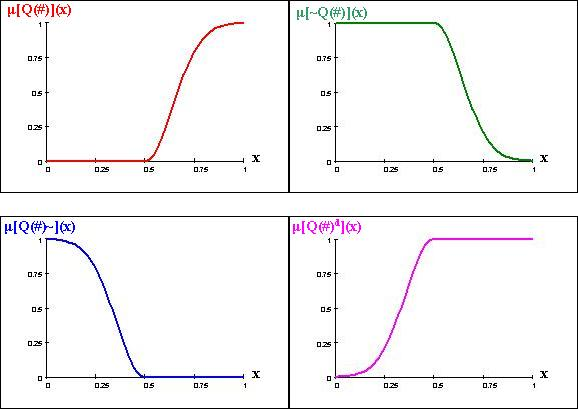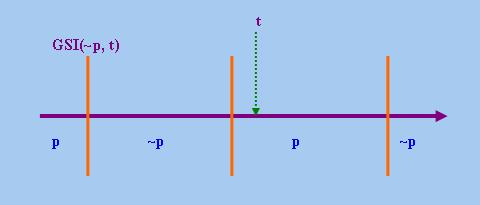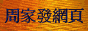# 廣義量詞系列：對偶性推理基礎

## 2. 基本概念

### 2.1 外部否定

some(A)
no(A)
(either John or Mary)(−)
(neither John nor Mary)(−)
(more than n)(A)
(at most n)(A)
(fewer than n)(A)
(at least n)(A)
(exactly n)(A)
(more or fewer than n)(A)
(between m and n)(A)
(more than n or fewer than m)(A)
(more than m but fewer than n)(A)
(at least n or at most m)(A)
(more than m of the n)(A)
(at most m of the n)(A)
(fewer than m of the n)(A)
(at least m of the n)(A)
(exactly m of the n)(A)
(more or fewer than m of the n)(A)
(between l and m of the n)(A)
(more than m or fewer than l of the n)(A)
(more than l but fewer than m of the n)(A)
(at least m or at most l of the n)(A)
(more than q)(A)
(at most q)(A)
(less than q)(A)
(at least q)(A)
(exactly q)(A)
(more or less than q)(A)
(between p and q)(A)
(more than q or less than p)(A)
(more than p but less than q)(A)
(at least q or at most p)(A)
(more ... than ...)(A, B)
(at most as many ... as ...)(A, B)
(fewer ... than ...)(A, B)
(at least as many ... as ...)(A, B)
(exactly as many ... as ...)(A, B)
(more or fewer ... than ...)(A, B)
(proportionally more ... than ...)(A, B)
(at most the same proportion of ... as ...)(A, B)
(proportionally fewer ... than ...)(A, B)
(at least the same proportion of ... as ...)(A, B)
(exactly the same proportion of ... as ...)(A, B)
(proportionally more or fewer ... than ...)(A, B)

### 2.2 內部否定

every(A)
no(A)
(both John and Mary)(−)
(neither John nor Mary)(−)
(exactly n)(A)
(all except n)(A)
(more than m of the n)(A)
(fewer than (n − m) of the n)(A)
(at least m of the n)(A)
(at most (n − m) of the n)(A)
(exactly m of the n)(A)
(exactly (n − m) of the n)(A)
(exactly m of the n)(A)
(all except m of the n)(A)
(between l and m of the n)(A)
(between (n − m) and (n − l) of the n)(A)
(more than l but fewer than m of the n)(A)
(more than (n − m) but fewer than (n − l) of the n)(A)
(at least m or at most l of the n)(A)
(at least (n − l) or at most (n − m) of the n)(A)
(more than m or fewer than l of the n)(A)
(more than (n − l) or fewer than (n − m) of the n)(A)
(more than q)(A)
(less than (1 − q))(A)
(at least q)(A)
(at most (1 − q))(A)
(exactly q)(A)
(exactly (1 − q))(A)
(exactly q)(A)
(all except q)(A)
(between p and q)(A)
(between (1 − q) and (1 − p))(A)
(more than p but less than q)(A)
(more than (1 − q) but less than (1 − p))(A)
(at least q or at most p)(A)
(at least (1 − p) or at most (1 − q))(A)
(more than q or less than p)(A)
(more than (1 − p) or less than (1 − q))(A)
(proportionally more ... than ...)(A, B)
(proportionally fewer ... than ...)(A, B)
(at least the same proportion of ... as ...)(A, B)
(at most the same proportion of ... as ...)(A, B)
(all except John)(A)
(no except John)(A)

### 2.3 對偶

∀(p) ≡ ~(∃(~p))
∃(p) ≡ ~(∀(~p))

every(A)
some(A)
(both John and Mary)(−)
(either John or Mary)(−)
(all except n)(A)
(more or fewer than n)(A)
(more than m of the n)(A)
(at least (n − m) of the n)(A)
(fewer than m of the n)(A)
(at most (n − m) of the n)(A)
(exactly (n − m) of the n)(A)
(more or fewer than m of the n)(A)
(all except m of the n)(A)
(more or fewer than m of the n)(A)
(between l and m of the n)(A)
(more than (n − l) or fewer than (n − m) of the n)(A)
(more than l but fewer than m of the n)(A)
(at least (n − l) or at most (n − m) of the n)(A)
(more than q)(A)
(at least (1 − q))(A)
(less than q)(A)
(at most (1 − q))(A)
(exactly (1 − q))(A)
(more or less than q)(A)
(all except q)(A)
(more or less than q)(A)
(between p and q)(A)
(more than (1 − p) or less than (1 − q))(A)
(more than p but less than q)(A)
(at least (1 − p) or at most (1 − q))(A)
(proportionally more ... than ...)(A, B)
(at least the same proportion of ... as ...)(A, B)
(proportionally fewer ... than ...)A, B)
(at most the same proportion of ... as ...)(A, B)

 ~Q(#)(P) ≡ ~(Q(#)(P)) (1) Q(#)~(P) ≡ Q(#)(~P) Q(#)d(P) ≡ ~(Q(#)(~P))

## 3. 普通<1>型量詞的對偶性推理

### 3.1 包含一層量詞語句的推理

 Q(#)(P) ⇔ It is not the case that ~Q(#)(P) (2) It is not the case that Q(#)(P) ⇔ ~Q(#)(P)

It is not the case that more boys than girls sang. ⇔ At most as many boys as girls sang.

 Q(#)(P) ⇔ Q(#)~(not P) (3) Q(#)(not P) ⇔ Q(#)~(P)

Exactly seven of the ten students smoke. ⇔ Exactly three of the ten students don't smoke.
Exactly 10% of John's friends didn't come. ⇔ All except 10% of John's friends came.

 Q(#)(P) ⇔ It is not the case that Q(#)d(not P) (4) It is not the case that Q(#)(P) ⇔ Q(#)d(not P) Q(#)(not P) ⇔ It is not the case that Q(#)d(P) It is not the case that Q(#)(not P) ⇔ Q(#)d(P)

Both John and Mary came. ⇔ It is not the case that either John or Mary did't come.

More or less than 10% of them don't smoke. ⇔ It is not the case that all except 10% of them smoke.

~(~(Q(#)(~(~P)))) ≡ Q(#)(P)

~~Q~~ ≡ Q

### 3.2 包含兩層量詞語句的推理

(Q ... Q')(#, #')(P)

Q(#)(Q'(#')(P)) (註4)

Q(#)(Q'(#')(P)) ⇔ Q(#)~(~Q'(#')(P))     (5)

Q(#)(~(~Q'(#')(P))) ≡ Q(#)(Q'(#')(P))

Q~~Q' ≡ QQ'

At least 2/3 of the students answered no question. ⇔ At most 1/3 of the students answered some question.
Every student except John read at least as many plays as poems. ⇔ No student except John read fewer plays than poems.
Both John and Mary sang at most two songs. ⇔ Neither John nor Mary sang more than two songs.

~Q~~Q'~ ≡ ~QQ'~

Q(#)d(Q'(#')d(P)) ⇔ ~Q(#)(Q'(#')~(P))     (6)

Every girl hates either John or Bill. ⇔ No girl hates neither John nor Bill.

### 3.3 包含三層量詞語句的推理

(Q ... Q' ... Q'')(#, #', #'')(P)

Q(#)(Q'(#')(Q''(#'')(P)))

Q(#)(Q'(#')(Q''(#'')(P))) ⇔ Q(#)~(Q'(#')d(~Q''(#'')(P)))     (7)

Q~~Q'~~Q'' ≡ QQ'Q''

Every family gave at least 40% of their old clothes to either organisation.
⇔ No family gave more than 60% of their old clothes to neither organisation.
Every family gave both organisations at least 40% of their old clothes.
⇔ No family gave either organisation less than 40% of their old clothes.

(~Q~)(Q')(~Q''~) ≡ (~Q)(~Q'~)(Q''~)

Q(#)d(Q'(#')(Q''(#'')d(P))) ⇔ ~Q(#)(Q'(#')d(Q''(#'')~(P)))     (8)

Some family gave at least 60% of their old clothes to either organisation.
⇔ Not every family gave more than 40% of their old clothes to neither organisation.

## 4. <1>型模糊量詞的對偶性推理

### 4.1 基本定義

μ[~Q(#)](x) = 1 − μ[Q(#)](x)

μ[Q(#)~](x) = μ[Q(#)](x')

x = |A ∩ P| / |A| − |B ∩ P| / |B|

 x' = |A ∩ ~P| / |A| − |B ∩ ~P| / |B| = |A − P| / |A| − |B − P| / |B|

μ[Q(#)~](x) = μ[Q(#)](1 − x)

μ[Q(#)d](x) = 1 − μ[Q(#)](1 − x)(almost every)(A)
(almost no)(A)
many(A)
few(A)
(a large proportion of)(A)
(a small proportion of)(A)
(a very large proportion of)(A)
(a very small proportion of)(A)
(a rather large proportion of)(A)
(a rather small proportion of)(A)
(much more than q)(A)
(much less than (1 − q))(A)
(slightly more than q)(A)
(slightly less than (1 − q))(A)
(approximately q)(A)
(approximately (1 − q))(A)
(proportionally far more ... than ...)(A, B)
(proportionally far fewer ... than ...)(A, B)

### 4.2 推理模式

Proportionally far more boys than girls did not show up. ⇔ Proportionally far fewer boys than girls showed up.

It is not the case that a very large proportion of the boys wear glasses.
⇔ Not a very small proportion of the boys do not wear glasses.

Many boys wear glasses. ⇔ It is not the case that not many boys wear glasses.
It is not the case that many boys wear glasses. ⇔ Not many boys wear glasses.

## 5. <1>型疑問量詞的對偶性推理

### 5.1 基本定義

∀a (World(Q(#)(P)(a)) ∩ World(~Q(#)(P)(a)) = Φ ∧ (World(Q(#)(P)(a)) ∪ World(~Q(#)(P)(a)) = W)

(more than how many)(A)
(at most how many)(A)
(fewer than how many)(A)
(at least how many)(A)

「疑問量詞」的「內部否定」的定義則可以沿用「陳述量詞」的「內部否定」的相應定義，即若Q(#)(P)為包含「<1>型疑問量詞」的語句，則其「內部否定」的形式為Q~(#)(P) ≡ Q(#)(~P)。在自然語言中，我們一般可以透過把疑問句的謂語轉換成其否定形式的方法來得到「疑問量詞」的「平凡內部否定」形式，但除了下表所示的「疑問量詞」外，絕大多數「<1>型疑問量詞」都沒有「非平凡內部否定」形式：

(proportionally how many more ... than ...)(A, B)
(proportionally how many more ... than ...)(B, A)

 C = |A − P| / |A| − |B − P| / |B| = 1 − |B − P| / |B| − 1 + |A − P| / |A| = (1 − |B − P| / |B|) − (1 − |A − P| / |A|) = |B ∩ P| / |B| − |A ∩ P| / |A|

(proportionally how many more ... than ...)(A, B)~ ≡ (proportionally how many more ... than ...)(B, A)

### 5.2 推理模式

Proportionally how many more boys than girls did not show up?
⇔ Proportionally how many more girls than boys showed up?

Proportionally how many more boys than girls have got a prize?
⇔ Proportionally how many more girls than boys have got no prize?

At least how many books did every boy read?     (9)

John read at least 3 books, Bill read at least 2 books and Peter read at least 5 books.

Which natural number n is such that every boy read at least n books?     (10)

Every boy read at least 2 books.

At least how many books did every boy read? ⇔ Fewer than how many books did no boy read?

## 6. 其他類型量詞的對偶性推理

### 6.1 數量比較詞

Proportionally more boys did not sing than girls not dance. ⇔ Proportionally fewer boys sang than girls danced.

Q1(S, S')(Q2(O)(P), Q3(O')(P'))

Q1(S, S')(Q2(O)(P), Q3(O')(P')) ⇔ Q1(S, S')~(~Q2(O)(P), ~Q3(O')(P'))     (13)

Q1(Q2, Q3) ≡ Q1(~~Q2, ~~Q3)

Proportionally more boys borrowed some books than girls bought at least 3 CDs.
⇔ Proportionally fewer boys borrowed no books than girls bought fewer than 3 CDs.

Every boy knows at least as many girls as some teacher does.     (14)

every(BOY)(([some(TEACHER)]2((at least as many ... as ...)(GIRL)(KNOW))

Every boy knows at least as many girls as some teacher does. ⇔ No boy knows fewer girls than every teacher does.

### 6.2 同異比較詞

(the same ... as ...)(A)(P, P')
differentw(A)(P, P')

Different boys sang than danced. ⇔ It is not the case that the same boys sang as danced.

No 2 boys answered the same questions.

no({X ⊆ BOY: |X| = 2})((the same)(QUESTION)(ANSWER))

No 2 boys answered the same questions. ⇔ Any 2 boys answered different questions.

At least 1/3 of the boys like the same singers as both Mary and Susan.

(at least 1/3)(BOY)(([(both Mary and Susan)(−)]2((the same ... as ...)(SINGER)(LIKE))

At least 1/3 of the boys like the same singers as both Mary and Susan.
⇔ At most 2/3 of the boys like different singers than either Mary or Susan.

## 7. 對偶性推理的推廣

### 7.1 其他論域

□(p) ≡ ~(◇(~p))
H(p) ≡ ~(P(~p))

John spent more time playing than studying yesterday.

John did not spend more time playing than studying yesterday.
⇔ John spent at most as much time playing as studying yesterday.

John is the first person in the queue.

every(QUEUE − {j})({x: (in front of)(Loc(j), Loc(x))})

John is the first person in the queue. ⇔ Nobody is in front of John in the queue.

John每逢進餐前都做祈禱。

every(HAVING-MEAL)({e': some(PRAYING)(SHORTLY-BEFORE−1e')})     (15)

John每逢進餐前都做祈禱。 ⇔ John不會在進餐前不做祈禱。

John met Mary in every library.

every(LIBRARY)({x: some(e: PROPOSITION(e) = MEET(j, m) ∧ inside(PLACE(e))(Loc(x))))(Power(T))})     (16)

John met Mary in every library. ⇔ In no library was Mary not met by John.

### 7.2 體貌副詞

Lobner在其論文Phase Quantification: A Uniform Treatment of Some Quantifiers from Different Categories以及Quantifications as a Major Module of Natural Language Semantics中提出了「階段量」(Phase Quantification)的概念，他把某些「體貌副詞」(Aspectual Adverb)的語義解釋成某種量化現象，以下筆者介紹這些副詞的對偶性以及相關的推理。already(p, t) ⇔ some({x: GSI(~p, t) < x ≤ t})({x: p(x)})

still(p, t) ⇔ every({x: GSI(p, t) < x ≤ t})({x: p(x)})
(not yet)(p, t) ⇔ no({x: GSI(~p, t) < x ≤ t})({x: p(x)})
(no longer)(p, t) ⇔ (not every)({x: GSI(p, t) < x ≤ t})({x: p(x)})

S Q V

S Q V ⇔ S Q~ ~V     (17)
S Qd V ⇔ S ~Q ~V     (18)

John仍然醒著。 ⇔ John尚未入睡。
John已經醒來。 ⇔ John不再睡著。

### 7.3 體貌動詞

Lobner還討論了四個「體貌動詞」(Aspectual Verb)：「繼續」("continue")、「不開始」("not begin")、「開始」("begin")和「停止」("stop")。從直觀上說，這四個動詞之間也存在各種否定關係，例如由於「停止不V」相當於「開始V」，可見「開始」與「停止」之間存在「內部否定」的關係。現把這四個動詞之間的關係總結成下表：

Lobner也嘗試把上述「體貌動詞」表達成量詞，不過他的表達方式其實隱含著多個預設，而且與前述有關「體貌副詞」的表達方式有很多不一致之處，所以這裡不擬列出(註6)。

John停止發聲。 ⇔ John開始沉默。
John繼續沉默。 ⇔ John不開始發聲。

### 7.4 條件句

Except when John is absent, otherwise if Bill sings then Mary will (surely) sing too.     (19)

If and only if John is absent, it is not the case that if Bill sings then Mary will sing too.

If John is absent, then (even) if Bill sings, Mary probably will not sing.
If John is not absent, then if Bill sings, Mary will (surely) sing too.

some(World(ABSENT(j)) ∩ World(SING(b)))(~World(SING(m)))
every(~World(ABSENT(j)) ∩ World(SING(b)))(World(SING(m)))

(except when ... otherwise)(p)(if(q)(r))     (20)

(except when ... otherwise)(p)(q) ⇔ (if and only if)(p)(~q)

~if(p)(q) ⇔ (probably … and)(p)(~q)

 (except when ... otherwise)(p)(if(q)(r)) ⇔ (if and only if)(p)(~if(q)(r)) ⇔ (if and only if)(p)((probably … and)(q)(~r))

Except when John is absent, otherwise if Bill sings then Mary will (surely) sing too.
⇔ If and only if John is absent, probably Bill sings but Mary does not sing.

### 7.5 原因句與讓步句

This house is no less comfortable because it dispenses with air-conditioning.
⇔ This house is no less comfortable, although it dispenses with air-conditioning.

~because(p)(q)     (21)
although(p)(~q)     (22)

Iten在Because and Although: a case of duality?一文中反對Konig的論點，她認為形如「because(p)(q)」的「原因句」的真值條件應為三個命題的合取：

p真 ∧ q真 ∧ p是q的原因

 (1) p真 ∧ q假 (2) p假 ∧ q真 (3) p假 ∧ q假 (4) p真 ∧ q真 ∧ p不是q的原因

|A ∩ ~P| = |A − P|<script type = "text/javascript"> (function(d, w) { var x = d.getElementsByTagName('SCRIPT'); var f = function() { var _id = 'lexity-pixel'; var _s = d.createElement('script'); _s.id = _id; _s.type = 'text/javascript'; _s.async = true; _s.src = "//np.lexity.com/embed/YW/be0aa169de7f441c6473361be62c9ef6?id=ddad453e7753"; if (!document.getElementById(_id)) { x.parentNode.insertBefore(_s, x); } }; w.attachEvent ? w.attachEvent('onload', f) : w.addEventListener('load', f, false); }(document, window)); </script>